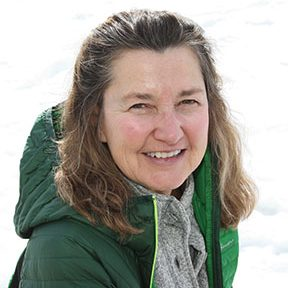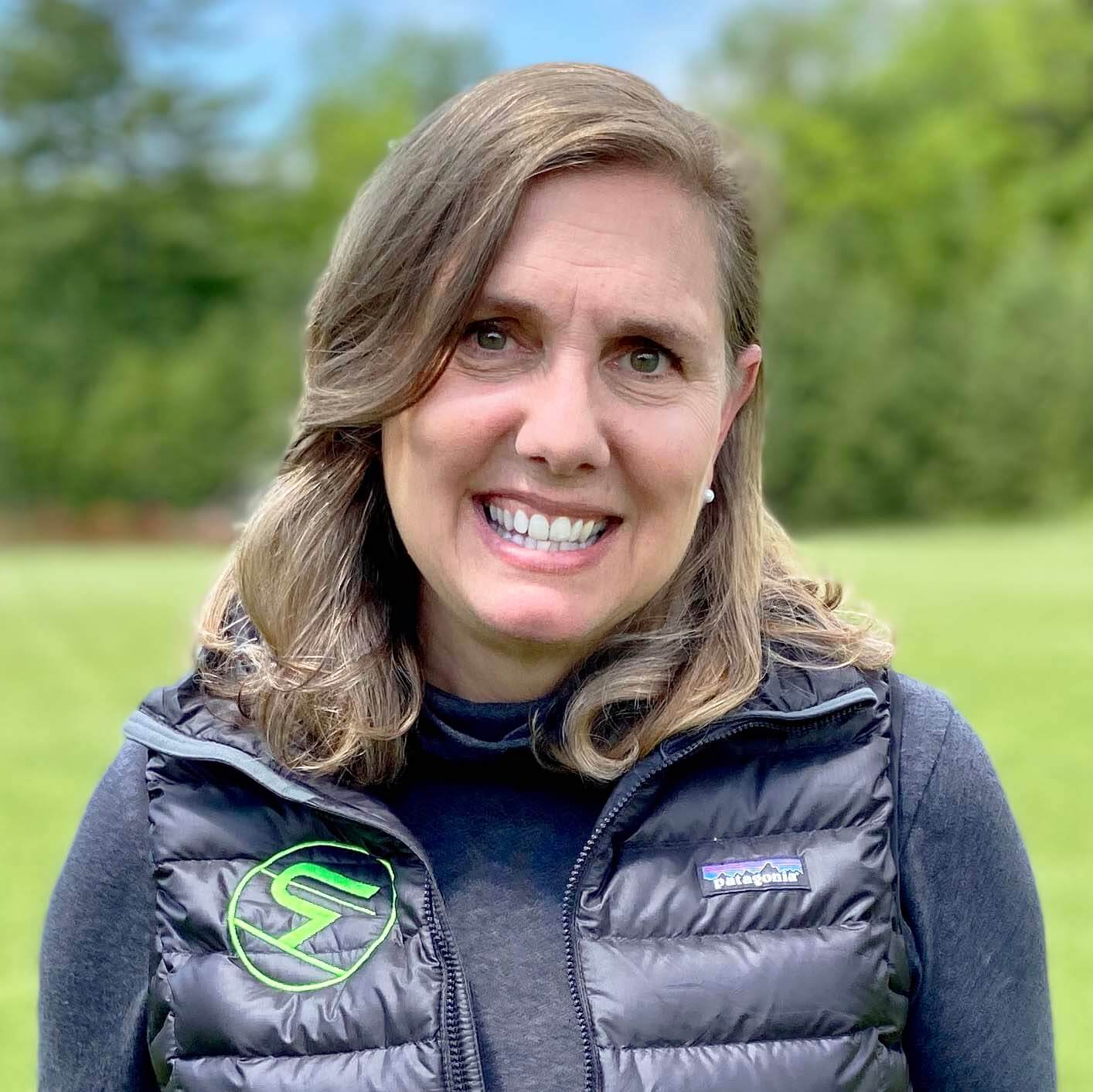# Departments & Curriculum Mathematics

Our mathematics department is staffed with knowledgeable and compassionate teachers who strive to build our students’ confidence and aptitude for math in all its forms. We offer a series of courses that build on each other to cover a broad base of mathematical concepts, ensuring that our students are equipped with the skills needed to be successful as they move through our math courses and beyond into college and the varied courses they will pursue.  Ultimately we aim to nurture mathematically confident students by teaching them to think logically and creatively, problem solve, communicate clearly and build a vast set of skills, tools and techniques for navigating life’s challenges.

## Courses

### Algebra 1

Algebra 1 is designed to develop core knowledge and skills. Students will use these skills in every subsequent mathematics course. Through the learning of algebra and its language of symbols, students learn to overcome challenge, learn to think critically, and to problem solve. This enables them to adapt, struggle and persevere by taking initiative in applying the skills creatively. Successful students discover the value of self-motivation because it is required to piece together knowledge and skill and thereby solve problems of various complexities. The particular algebra skills learned include simplifying rational expressions and radicals, factoring polynomials, solving equations and inequalities, and graphing linear functions in two variables.

### Geometry

This is a comprehensive course in Geometry that develops a student’s ability to reason mathematically, try new ideas, and solve problems. Logical reasoning and spatial visualization skills, as well as real world applications are emphasized. Deductive reasoning is used to solve problems utilizing the formal two column proof method. The course includes regular algebra review.

### Algebra 2

This course is designed for students who will benefit greatly from an extensive review of topics covered in Algebra 1, but who also desire to explore additional subjects typical of an Algebra 2 course. Most students continue to pre-calculus. Class time consists of lectures and concept demonstrations by the instructor; however, an emphasis is placed on students working problems to completion independently and in groups. Students reflect on the process by writing a sentence or two affirming the concepts or key principles involved in approaching and solving the problem at hand.

### Pre Calculus

Seeing the link between numbers and symbols in an equation and the graph of this equation on a Cartesian plane is the overarching goal of this course. In the process we solidify our algebra skills in the context of understanding how the numbers transform the pictures of a dozen function types. The numbers change the picture of the function in conceptual ways and when those are understood, the nuances become far easier to clarify. We spend a majority of class time learning polynomial, rational, exponential, logarithmic, and the six trigonometric functions. We also investigate how inverses work both algebraically and visually. We begin the year revisiting quadratics and increase the rigor by using the power of these functions to perform in-depth data modeling and applying the functions to solve problems using real data. This focus on applying understanding to word problems with the aid of a graphing calculator makes this a very challenging course. As a result, a successful student will be prepared for calculus and empowered to excel at the college level.

### Probability & Statistics

Probability, Statistics, Big Data, Data Analytics, Machine Learning and Game Theory are changing the way we interface with the world to a greater degree than most people realize. In this course, we will learn the foundational principles and theories that drive data applications and examine systems. Intended as a follow-up to Algebra II or an equivalent course, our course is designed to complete the study of the elementary functions (linear, quadratic, exponential, logarithmic, and trigonometric). We will cover the process of building prediction functions including data collection, interpretation and evaluation with an emphasis on practical applications. The main goal of the course is to equip students with the tools they need to understand and apply data analytics into the future.

### Calculus

Calculus is frequently described as the mathematics of change. Simultaneously invented/discovered by separate individuals in the 17th century, calculus is a mathematical tool that allows for an in depth analysis of mathematical functions that are used to model real world behavior. Students taking this course should have successfully completed Pre Calculus and should have familiarity using a graphing calculator. Throughout the course, students are encouraged and expected to solve problems both graphically (with the aid of a graphing calculator) and analytically (pencil and paper). Although it’s a math course, students will be asked frequently to verbally explain the concept behind the solutions they find, and on tests they will be frequently asked to write a sentence or two to justify their answers. Students will be presented with comprehensive AP Calculus problems, and at the end of the year, all students will be expected to take a cumulative exam.

## Meet Our Mathematics Faculty### Dave Beningson

Science and Math Teacher### Laura Gailiunas

Math and French Teacher, Learning Services Support### Andrea Harris

Dean of College Counseling, History/Math Teacher